# How to Calculate and Solve for Fluid Potential, Pressure, Datum Level and Density | The Calculator Encyclopedia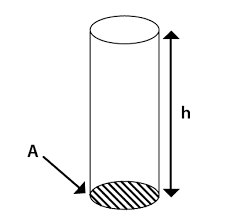The image above represents the fluid potential.

To compute for the fluid potential, three essential parameters are needed and these parameters are pressure (P), Datum Levels (ΔZ) and Density (ρ).

The formula for calculating fluid potential:

φ = P – [ρ / 144]ΔZ

Where;
φ = Fluid Potential
P = Pressure
ΔZ = Datum Levels
ρ = Density

Let’s solve an example;
Find the fluid potential when the pressure is 24 with a datum level of 18 and the density of 30.

This implies that;
P = Pressure = 24
ΔZ = Datum Levels = 18
ρ = Density = 30

φ = P – [ρ / 144]ΔZ
φ = 24 – [30/144] 18
φ = 24 – [0.2083] 18
φ = 24 – 3.75
φ = 20.25

Therefore, the fluid potential is 20.25.

Calculating the Pressure(P) when the fluid potential, Datum levels and Density is Given.

P = φ – [ρ / 144]ΔZ

Where;
P = Pressure
φ = Fluid Potential
ΔZ = Datum Levels
ρ = Density

Lets solve an example;
Find the pressure with a fluid potential of 40 and a datum levels of 18 with density of 24.

This implies that;
φ = Fluid Potential = 40
ΔZ = Datum Levels = 18
ρ = Density = 24

P = φ – [ρ / 144]ΔZ
P = 40 – [24 / 144]18
P = 40 – [0.167]18
P = 40 – 3
P = 37

Therefore, the pressure is 37.

Calculating the Datum levels(ΔZ) when the fluid potential, Pressure and Density is Given.

ΔZ = (θ – P) 144 / p

Where;
ΔZ = Datum Levels
φ = Fluid Potential
P = Pressure
ρ = Density

Let’s solve an example;
Find the datum levels with a fluid potential of 50, a pressure of 25 and density of 14.

This implies that;
φ = Fluid Potential = 50
P = Pressure = 25
ρ = Density = 14

ΔZ = (θ – P) 144 / p
ΔZ = (50 – 25) 144 / 14
ΔZ = (25) 144 / 14
ΔZ = 3600 / 14
ΔZ = 257.14

Therefore, the datum levels is 257.14.

Calculating the Density (p) when the fluid potential, Pressure and Datum levels is Given.

p = (θ – P) 144 / ΔZ

Where;
ρ = Density
ΔZ = Datum Levels
φ = Fluid Potential
P = Pressure

Let’s solve an example;
Find the density with a fluid potential of 62, pressure of 36 and datum levels of 24.

This implies that;
φ = Fluid Potential = 62
ΔZ = Datum Levels = 24
P = Pressure = 36

p = (θ – P) 144 / ΔZ
p = (62 – 36) 144 / 24
p = (26) 144 / 24
p = 3744 / 24
p = 156

Therefore, the density is 156.

Nickzom Calculator – The Calculator Encyclopedia is capable of calculating the fluid potential.

To get the answer and workings of the fluid potential using the Nickzom Calculator – The Calculator Encyclopedia. First, you need to obtain the app.

You can get this app via any of these means:

To get access to the professional version via web, you need to register and subscribe for NGN 1,500 per annum to have utter access to all functionalities.
You can also try the demo version via https://www.nickzom.org/calculator

Apple (Paid) – https://itunes.apple.com/us/app/nickzom-calculator/id1331162702?mt=8
Once, you have obtained the calculator encyclopedia app, proceed to the Calculator Map, then click on Petroleum under EngineeringNow, Click on Reservoir Fluid Flow under Petroleum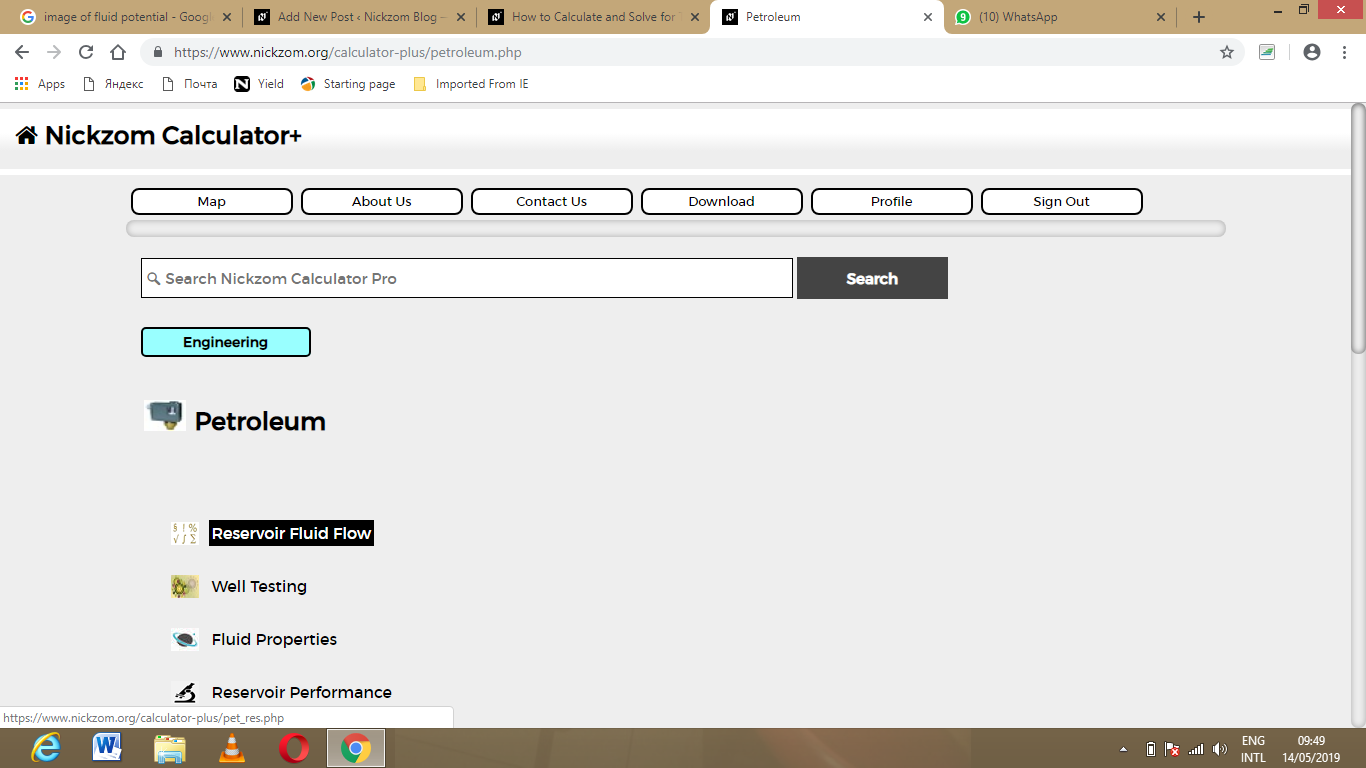Now, Click on Fluid Potential under Reservoir Fluid Flow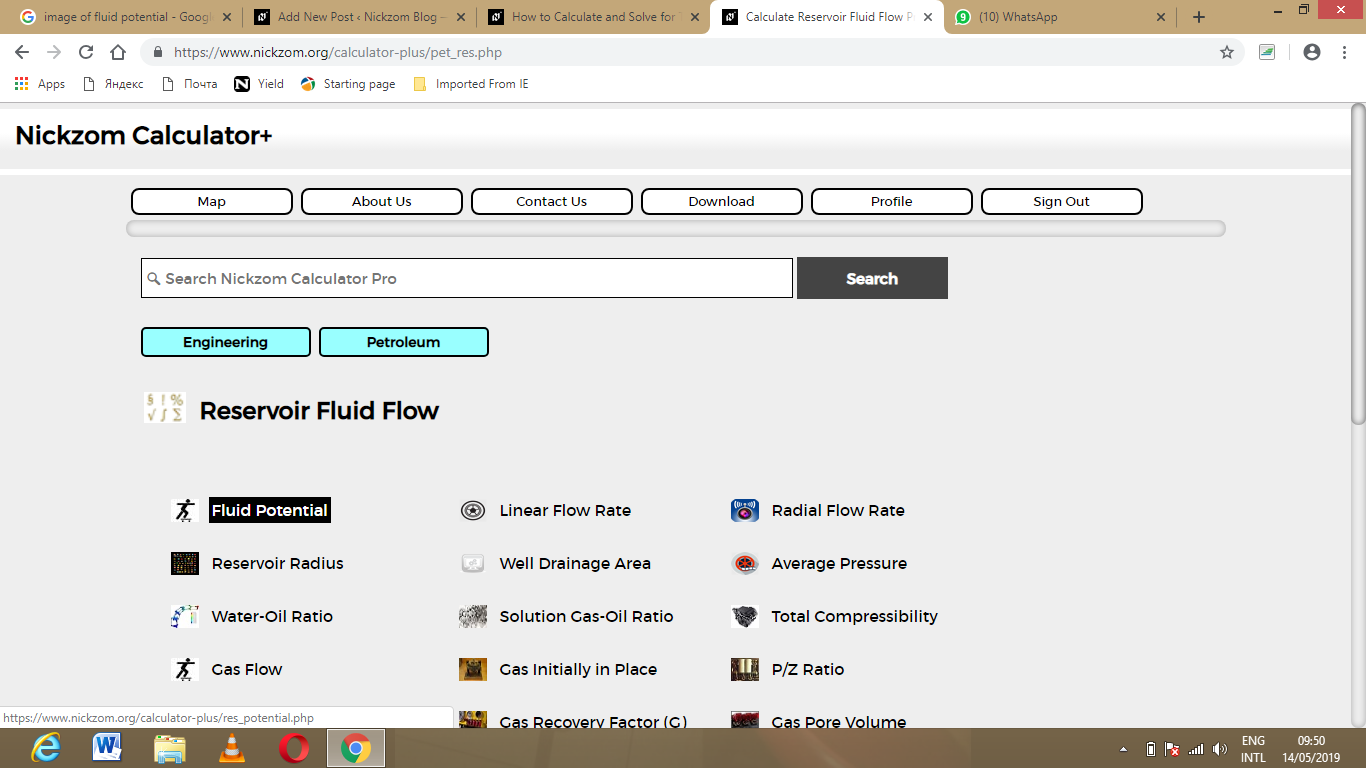The screenshot below displays the page or activity to enter your value, to get the answer for the fluid potential according to the respective parameter which are the pressure (P), Datum Levels (ΔZ) and Density (ρ).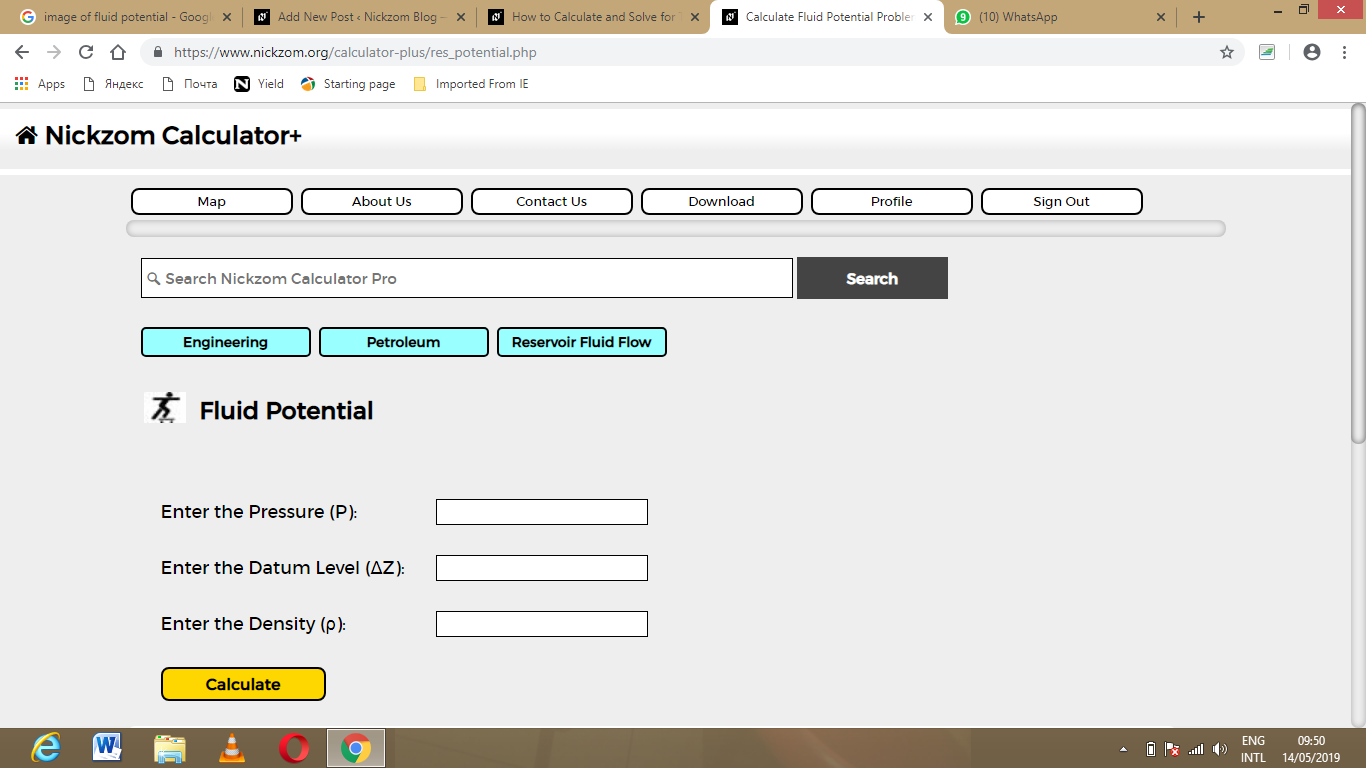Now, enter the value appropriately and accordingly for the parameter as required by the pressure (P) is 24, Datum Levels (ΔZ) is 18 and Density (ρ) is 30.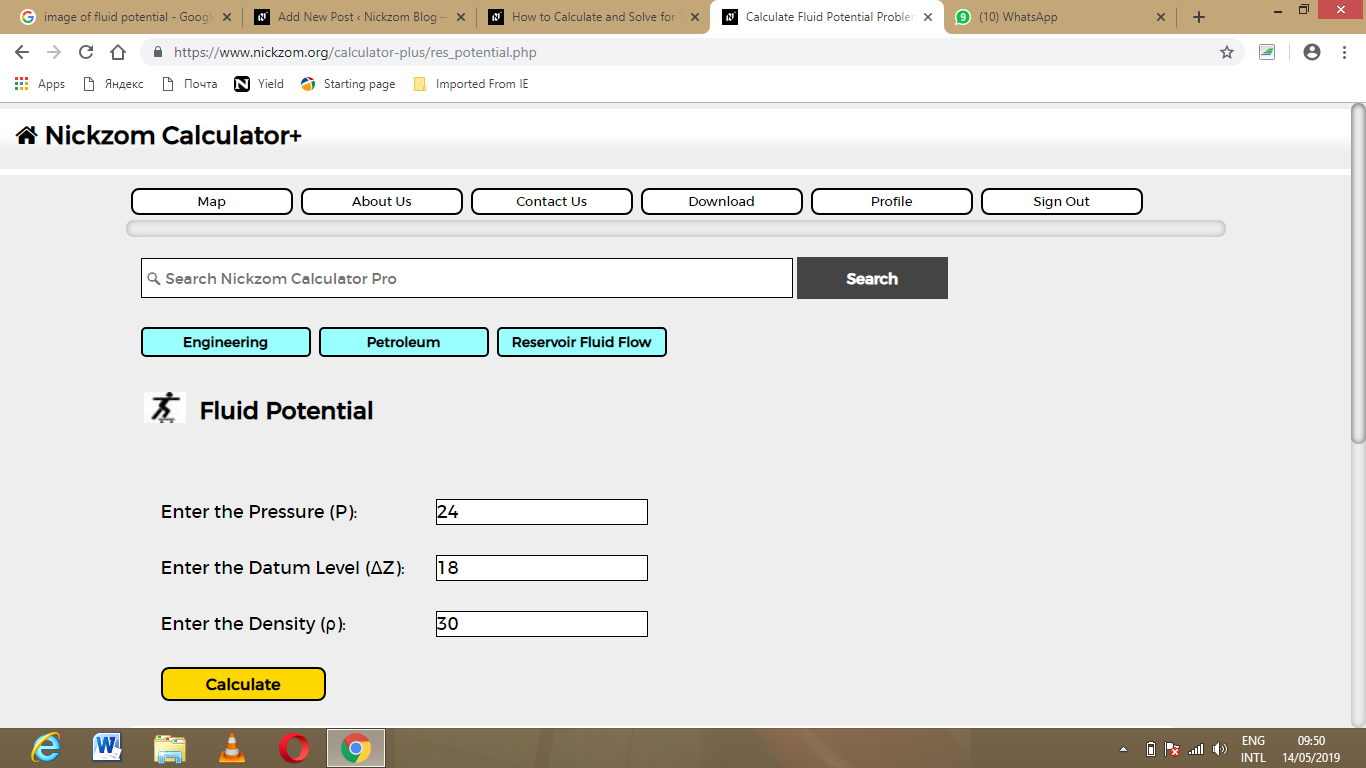Finally, Click on Calculate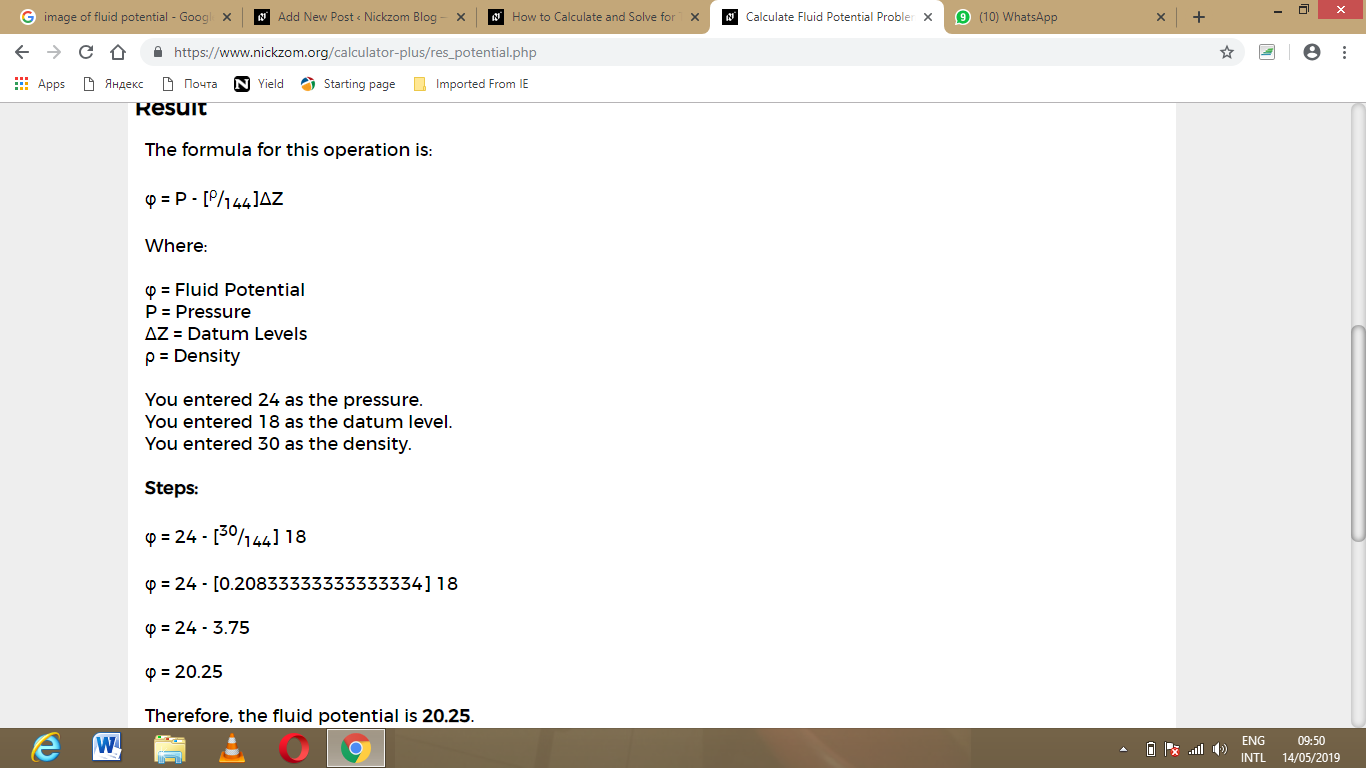As you can see from the screenshot above, Nickzom Calculator – The Calculator Encyclopedia solves for the fluid potential and presents the formula, workings and steps too.